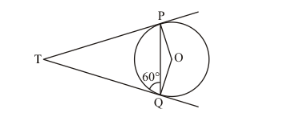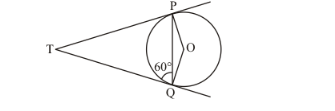# In the given figure, if TP and TQ are tangents drawn from an external point

Question:

In the given figure, if TP and TQ are tangents drawn from an external point T to a circle with centre O such that ∠TQP = 60°, then ∠OPQ =(a) 25°

(b) 30°

(c) 40°

(d) 60°

Solution:

Consider.We have,

TP = TQ(Tangents from an external point will be equal)

We know that angles opposite to equal sides will be equal. Therefore,

$\angle T Q P=\angle T P Q$

It is given that,

$\angle T Q P=60^{\circ}$

Therefore,

$\angle T P Q=60^{\circ}$

We know that the radius will always be perpendicular to the tangent at the point of contact. Therefore,

$O P \perp P T$

Hence,

$\angle T P O=90^{\circ}$

That is,

$\angle T P Q+\angle Q P O=90^{\circ}$

We have found that,

$\angle T P Q=60^{\circ}$

Therefore,

$60^{\circ}+\angle Q P O=90^{\circ}$

$\angle Q P O=30^{\circ}$

Therefore, the correct answer is option (b).